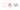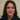# Build a clock with Javascript and CSS using CSS transform

We will build a clock with Javascript and CSS transform and the Date() function. The original idea is from wesbos.com  which has nice Javascript ideas for all. I have created a pen for it and I will explain it as it is. This is the pen:

We have three divs for seconds and minutes and hours by the class of “hand”. We will explain the most important part of the clock here. So without the Javascript and the transform line of code in the .hand class in CSS this is what you will see:All three hands are above each other pointing to the nine it seams. So first we need to make them point to twelve where the clock actually starts.

### The transform code

This is where the transform comes in:

`transform-origin: 100%;`
`transform: rotate(90deg);`

We change the transform-origin from 50% which is its default value to 100%. Because we need to make the hands to turn inside the clock and their pin point will stick to the middle of the clock. So we change the x axis point to be started from the very middle of the clock.

However this is not enough. Although it will ake the hands to turn pointing right to the middle but they do not start from twelve ‘o clock. They are stuck to nine ‘o clock at the moment.Thus we rotate them by 90 degrees and that will do the trick:We have the clock ready now. Lets add the Javascript. As always we get the elements we need to work on:

`const secondHand = document.querySelector('.second-hand'); // the seconds hand pointer`
`const minsHand = document.querySelector('.min-hand'); // the minutes hand pointer`
`const hourHand = document.querySelector('.hour-hand'); // the hour hand pointer`

Great. All set. We have to write a function to create our clock behaviour and run it every second:

`setInterval(setDate, 1000);`

We need to write the seDate function. First we will get the seconds from the Date function:

`const now = new Date();`
`const seconds = now.getSeconds();`

We need to turn this into degrees so that we could use transform in CSS to update the seconds hand.

`const secondsDegrees = ((seconds / 60) * 360) ;`

This will give us what we want. We divide the seconds by 60 which gives a percentage and as transform has 360 degrees , we multiply it by then.But the problem is because we add a 90 degree offset to our hand in the beginning, each second will have 15 seconds offset as well if that makes sense. Therefor we need to add 90 degree offset to the code to make it work:

`const secondsDegrees = ((seconds / 60) * 360) + 90;`

Now we update the transform property of our seconds hand using the ES6 template literals syntax:

`secondHand.style.transform = `rotate(\${secondsDegrees}deg)`;`

And this gives us the seconds for the clock.

Same thing applies for the minutes hand:

`const mins = now.getMinutes();`
`const minsDegrees = ((mins / 60) * 360) + 90;`
`minsHand.style.transform = `rotate(\${minsDegrees}deg)`;`

And for the hours hand as well:

`const hour = now.getHours();`
`const hourDegrees = ((hour / 12) * 360) + 90;`
`hourHand.style.transform = `rotate(\${hourDegrees}deg)`;`

All done. With a few lines of code we built a clock and combining transform with the Date function. We will build a modern clock with Javascript and react later on in a seperate post. But this was to show a simple approach to use the basic techniques.